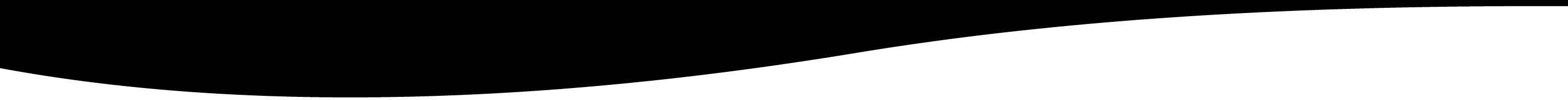# continue

Stop execution of a loop and continue for the next iteration.

# CONTINUE

## NAME

continue - Stops execution of a loop and continues for the next iteration.

continue;

## DESCRIPTION

This statement is used to stop the execution of a loop and to continue to the next iteration. This can be used with For, While, or Do Until. Here is an example:

```for(i=0,j=4;i<5;++i,--j) do
i;
j;
if i==2 then continue; endif
'i=';
i;
endfor return 04i=013i=12231i=340i=4
```

The continue stop the execution and goes to the next increment of the loop.

## EXAMPLES

Note: In the followings examples, the _ between the { should be removed to make it work.

```{_{
function f(i)
"i="; i;
sn_var = i*2;
return i>5;
endfunction

k=10;
for j f(k) do
"j="; j;
k--;
if (k==8) then
continue;
endif
';';
endfor
}}

return i=10j=20;i=9j=18i=8j=16;i=7j=14;i=6j=12;i=5
```